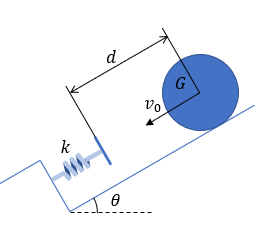# A cylinder with a mass m = 20 kg and radius r = 0.5 m is rolled down a rough surface toward a...

## Question:

A cylinder with a mass m = 20 kg and radius r = 0.5 m is rolled down a rough surface toward a spring of stiffness k = 2000 N/m. the surface is angled at 30 degrees. The cylinder?s mass center G is initially d = 2 m from the spring. The cylinder rolls without slip down the incline. Find the initial speed {eq}{v}_{0} {/eq} of G if the compression of the spring is x = 0.5 m.## Rotational Kinetic Energy

Just as a body that is moving in pure translation has a kinetic energy associated with it, a body that is moving with a rotational motion also has a kinetic energy associated with it. This is referred to as rotational kinetic energy. It is dependent on the moment of inertia and angular velocity of the body.

The cylinder has an initial potential energy and kinetic energy which is then converted into spring energy. The initial kinetic energy of the cylinder is from translation as well as rotation.

Let us consider the maximum compression of the spring to be the reference plane. Using this reference plane, initial the height of the cylinder is,

{eq}h = (d + x)sin \theta \\ h = (2 + 0.5) sin30° = 1.25 \ m {/eq}

Applying the law of conservation of energy,

{eq}\displaystyle \frac {1}{2}kx^2 = mgh + \frac{1}{2}mv_o^2 + \frac{1}{2} I \omega_o^2 {/eq}

where moment of inertia of cylinder, {eq}\displaystyle I = \frac {1}{2}mr^2 {/eq} and angular velocity, {eq}\displaystyle \omega_o = \frac {v_o}{r} {/eq}.

Substituting these values in the above equation we get,

{eq}\displaystyle \frac {1}{2}kx^2 = mgh + \frac{1}{2}mv_o^2 + \frac{1}{2} \left (\frac{1}{2}mr^2 \right ) \left (\frac{v_o}{r} \right )^2 \\ \displaystyle \frac{1}{2}kx^2 = mgh + \frac{3}{4}mv_o^2 {/eq}

Now let us substitute the known values in the equation,

{eq}\displaystyle \frac{1}{2}*2000*(0.5)^2 = 20*9.81*1.25 + \frac{3}{4}*20*v_o^2 \\ v_o = 0.562 \ m/s {/eq}

This is the initial velocity of the cylinder.